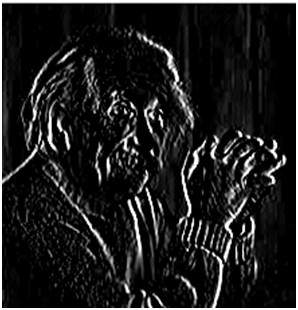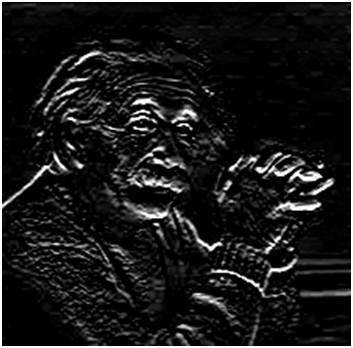# Sobel Operator

The sobel operator is very similar to Prewitt operator. It is also a derivate mask and is used for edge detection. Like Prewitt operator sobel operator is also used to detect two kinds of edges in an image:

• Vertical direction
• Horizontal direction

## Difference with Prewitt Operator

The major difference is that in sobel operator the coefficients of masks are not fixed and they can be adjusted according to our requirement unless they do not violate any property of derivative masks.

### Following is the vertical Mask of Sobel Operator:

 -1 0 1 -2 0 2 -1 0 1

This mask works exactly same as the Prewitt operator vertical mask. There is only one difference that is it has “2” and “-2” values in center of first and third column. When applied on an image this mask will highlight the vertical edges.

### How it works

When we apply this mask on the image it prominent vertical edges. It simply works like as first order derivate and calculates the difference of pixel intensities in a edge region.

As the center column is of zero so it does not include the original values of an image but rather it calculates the difference of right and left pixel values around that edge. Also the center values of both the first and third column is 2 and -2 respectively.

This give more weight age to the pixel values around the edge region. This increase the edge intensity and it become enhanced comparatively to the original image.

### Following is the horizontal Mask of Sobel Operator

 -1 -2 -1 0 0 0 1 2 1

Above mask will find edges in horizontal direction and it is because that zeros column is in horizontal direction. When you will convolve this mask onto an image it would prominent horizontal edges in the image. The only difference between it is that it have 2 and -2 as a center element of first and third row.

### How it works

This mask will prominent the horizontal edges in an image. It also works on the principle of above mask and calculates difference among the pixel intensities of a particular edge. As the center row of mask is consist of zeros so it does not include the original values of edge in the image but rather it calculate the difference of above and below pixel intensities of the particular edge. Thus increasing the sudden change of intensities and making the edge more visible.

Now it’s time to see these masks in action:

### Sample Image

Following is a sample picture on which we will apply above two masks one at time.After applying vertical mask on the above sample image, following image will be obtained.After applying horizontal mask on the above sample image, following image will be obtained### Comparison

As you can see that in the first picture on which we apply vertical mask, all the vertical edges are more visible than the original image. Similarly in the second picture we have applied the horizontal mask and in result all the horizontal edges are visible.

So in this way you can see that we can detect both horizontal and vertical edges from an image. Also if you compare the result of sobel operator with Prewitt operator, you will find that sobel operator finds more edges or make edges more visible as compared to Prewitt Operator.

This is because in sobel operator we have allotted more weight to the pixel intensities around the edges.

### Applying more weight to mask

Now we can also see that if we apply more weight to the mask, the more edges it will get for us. Also as mentioned in the start of the tutorial that there is no fixed coefficients in sobel operator, so here is another weighted operator

 -1 0 1 -5 0 5 -1 0 1

If you can compare the result of this mask with of the Prewitt vertical mask, it is clear that this mask will give out more edges as compared to Prewitt one just because we have allotted more weight in the mask.